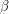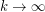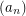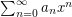# Rüdinger, Andreas

## Asymptotic Distribution of Form of Polyhedra ★★

Author(s): Rüdinger

Problem   Consider the set of all topologically inequivalent polyhedra withedges. Define a form parameter for a polyhedron aswhereis the number of vertices. What is the distribution offor?

Keywords: polyhedral graphs, distribution

## Criterion for boundedness of power series ★

Author(s): Rüdinger

Question   Give a necessary and sufficient criterion for the sequenceso that the power seriesis bounded for all.

Keywords: boundedness; power series; real analysis